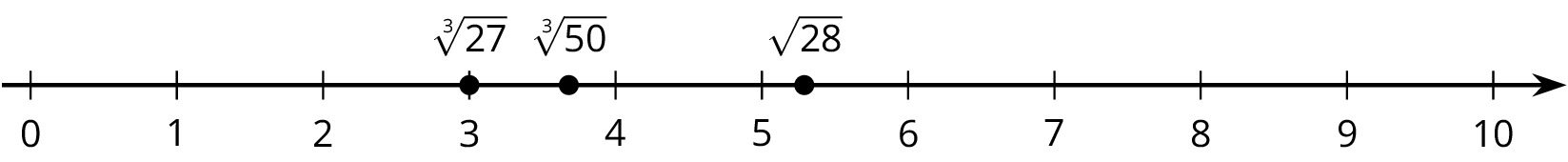## The Size of Shapes

This week your student will be working with the relationship between the side length and area of squares. We know two main ways to find the area of a square:

• Multiply the square’s side length by itself.
• Decompose and rearrange the square so that we can see how many square units are inside. For example, if we decompose and rearrange the tilted square in the diagram, we can see that its area is 10 square units.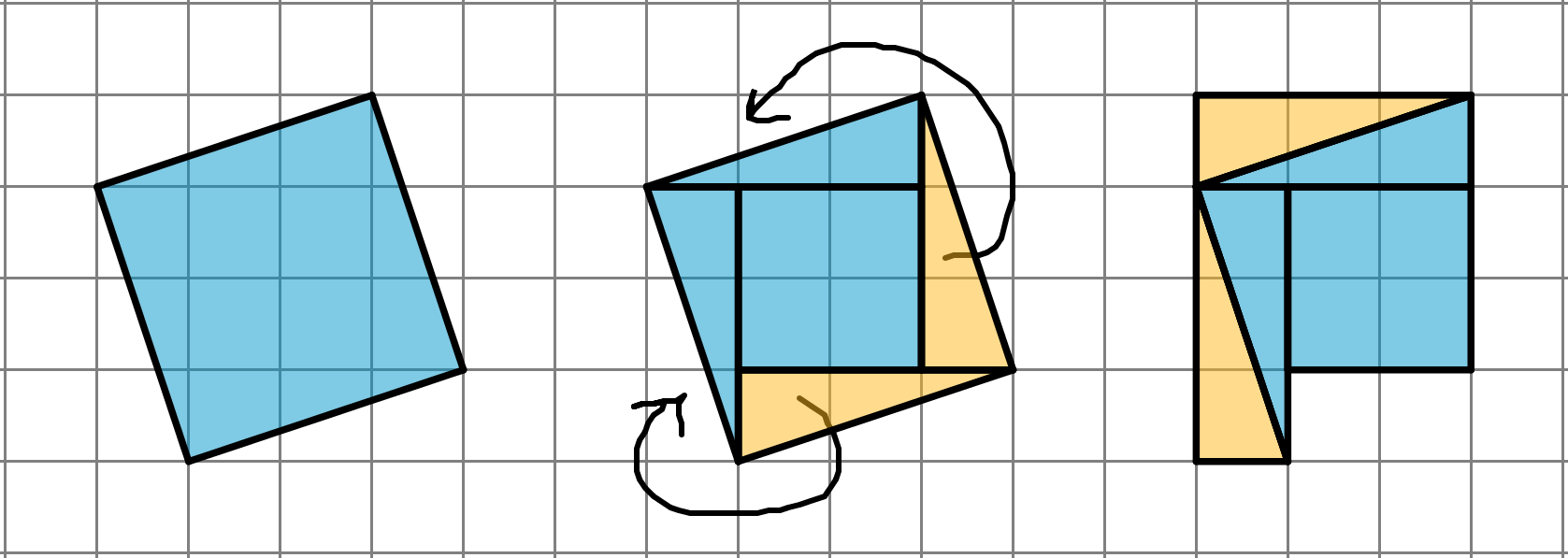But what is the side length of this tilted square? It cannot be 3 units since $3^2=9$ and it cannot be 4 units since $4^2=16$. In order to write “the side length of a square whose area is 10 square units,” we use notation called a square root. We write “the square root of 10” as $\sqrt{10}$ and it means “the length of a side of a square whose area is 10 square units.” All of these statements are true:

• $\sqrt{9}=3$ because $3^2=9$
• $\sqrt{16}=4$ because $4^2=16$
• $\sqrt{10}$ is the side length of a square whose area is 10 square units, and $\left(\sqrt{10}\right)^2=10$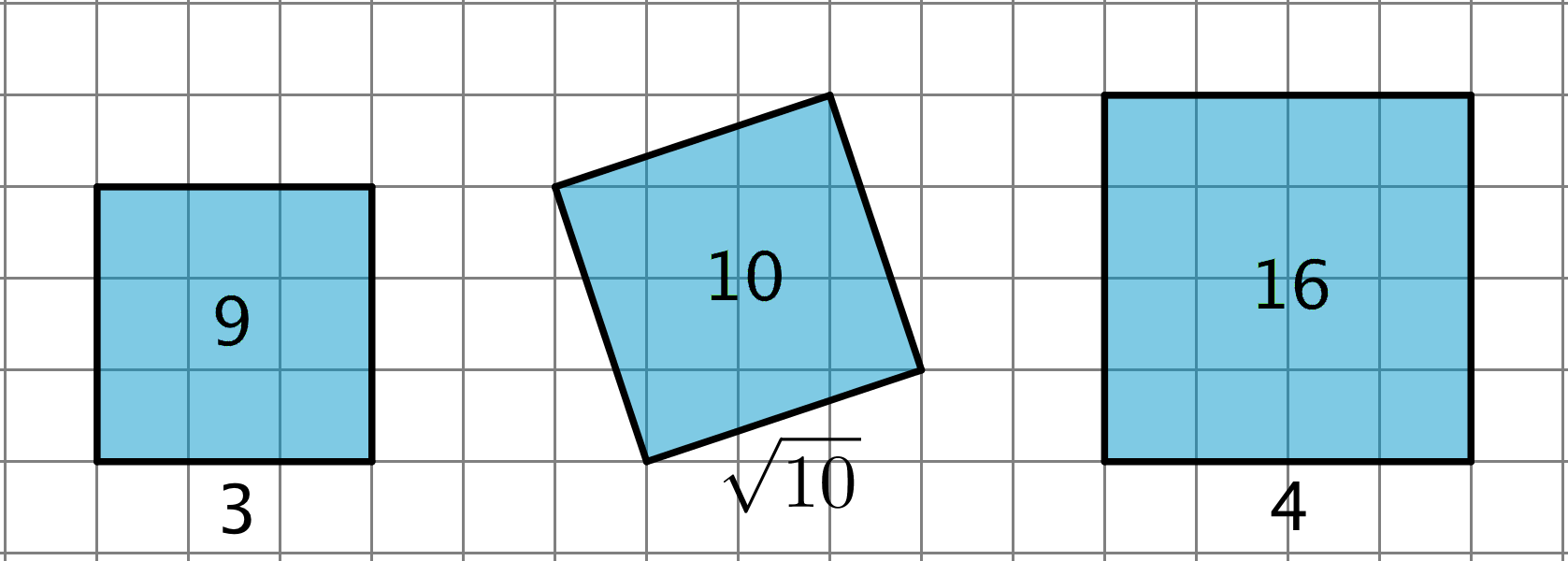If each grid square represents 1 square unit, what is the side length of this titled square? Explain your reasoning.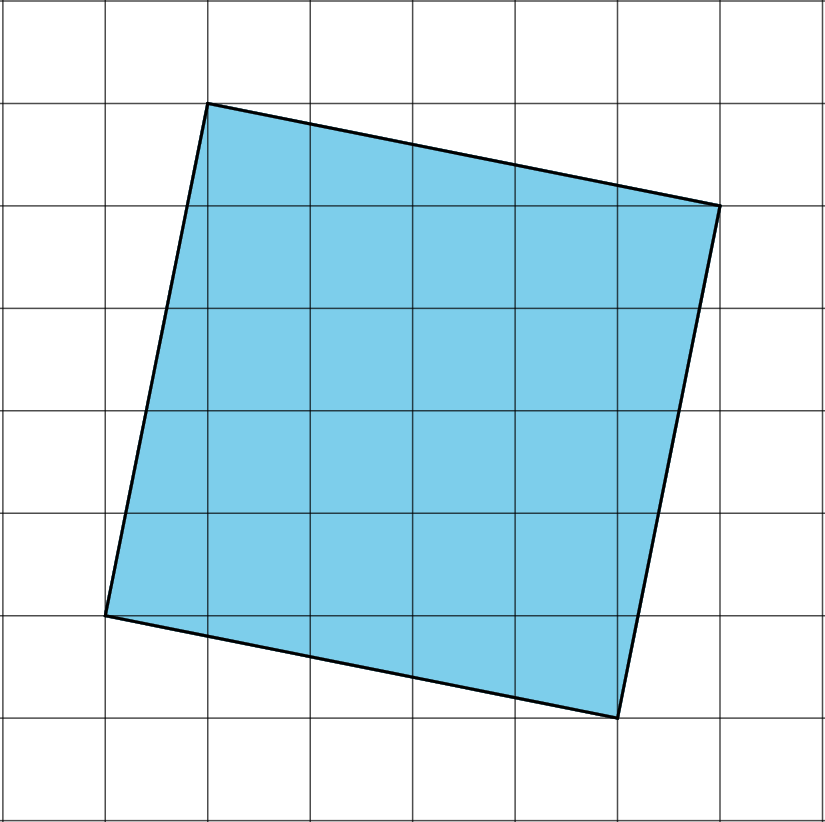Solution:

The side length is $\sqrt{26}$ because the area of the square is 26 square units and the square root of the area of a square is the side length.

## The Pythagorean Theorem

This week your student will work with the Pythagorean Theorem, which describes the relationship between the sides of any right triangle. A right triangle is any triangle with a right angle. The side opposite the right angle is called the hypotenuse, and the two other sides are called the legs. Here we have a triangle with hypotenuse $c$ and legs $a$ and $b$. The Pythagorean Theorem states that for any right triangle, the sum of the squares of the legs are equal to the square of the hypotenuse. In other words, $a^2+b^2=c^2$.We can use the Pythagorean Theorem to tell if a triangle is a right triangle or not, to find the value of one side length of a right triangle if we know the other two, and to answer questions about situations that can be modeled with right triangles. For example, let’s say we wanted to find the length of this line segment: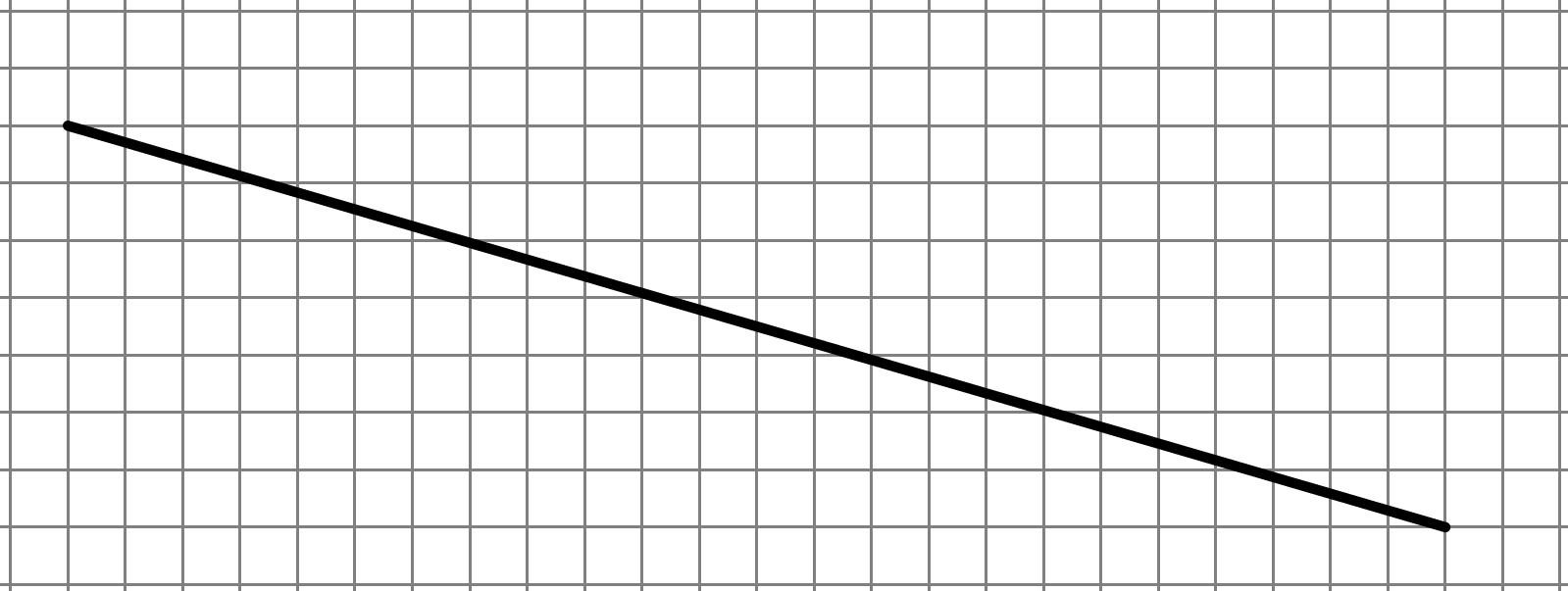We can first draw a right triangle and determine the lengths of the two legs: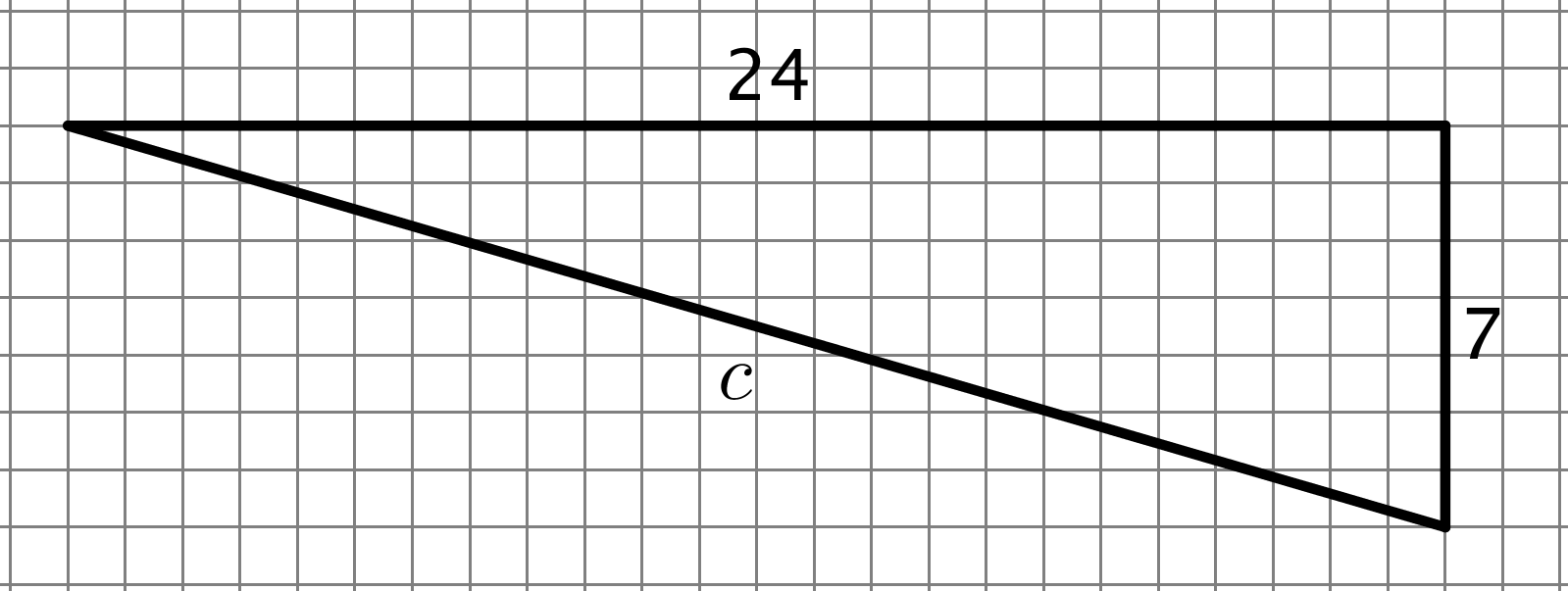Next, since this is a right triangle, we know that $24^2+7^2=c^2$, which means the length of the line segment is 25 units.

1. Find the length of the hypotenuse as an exact answer using a square root.2. What is the length of line segment $p$? Explain or show your reasoning. (Each grid square represents 1 square unit.)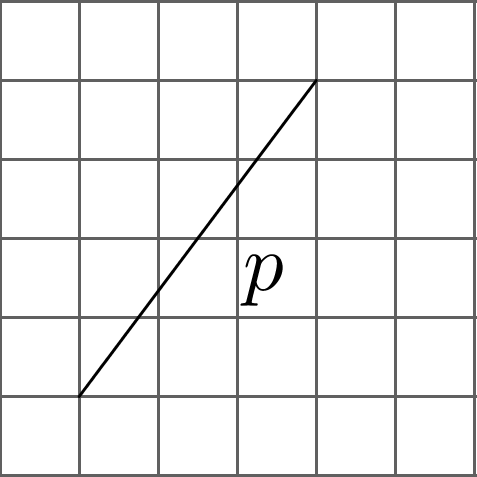Solution:

1. The length of the hypotenuse is $\sqrt{50}$ units. With legs $a$ and $b$ both equal to 5 and an unknown value for the hypotenuse, $c$, we know the relationship $5^2+5^2=c^2$ is true. That means $50=c^2$, so $c$ must be $\sqrt{50}$ units.
2. The length of $p$ is $\sqrt{25}$ or 5 units. If we draw in the right triangle, we have legs of length 3 and 4 and hypotenuse $p$, so the relationship $3^2+4^2=p^2$ is true. Since $3^2+4^2=25=p^2$, $p$ must equal $\sqrt{25}$ or 5 units.

## Side Lengths and Volumes of Cubes

This week your student will learn about cube roots. We previously learned that a square root is the side length of a square with a certain area. For example, if a square has an area of 16 square units then its edge length is 4 units because $\sqrt{16}=4$. Now, think about a solid cube. The cube has a volume, and the edge length of the cube is called the cube root of its volume. In this diagram, the cube has a volume of 64 cubic units: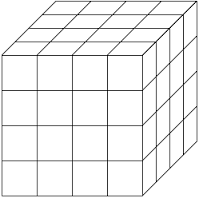Even without the useful grid, we can calculate that the edge length is 4 from the volume since $\sqrt{64}=4$.

Cube roots that are not integers are still numbers that we can plot on a number line. If we have the three numbers $\sqrt{40}$, $\sqrt{30}$, and $\sqrt{64}$, we can plot them on the number line by estimating what integers they are near. For example, $\sqrt{40}$ is between 6 and 7, since $\sqrt{36}<\sqrt{40}<\sqrt{49}$ and $\sqrt{36}=6$ while $\sqrt{49}=7$. Similarly, $\sqrt{30}$ is between 3 and 4 because 30 is between 27 and 64. Our number line will look like this: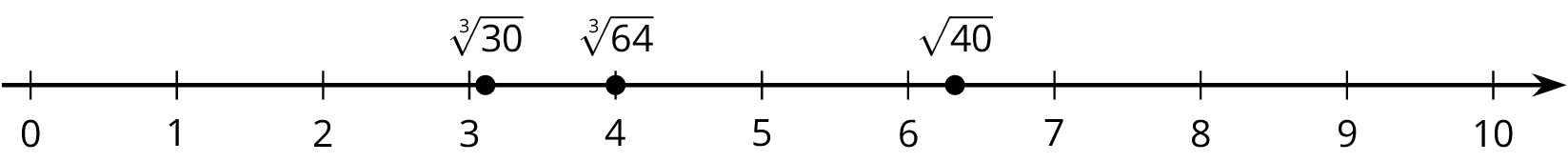Plot the given numbers on the number line: $\sqrt{28}$, $\sqrt{27}$, $\sqrt{50}$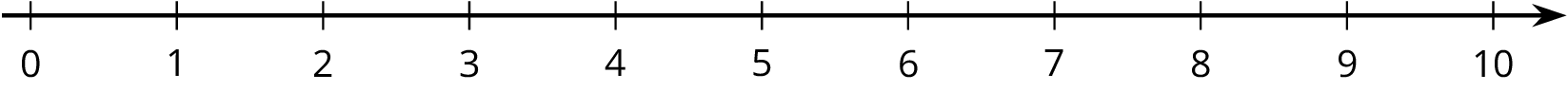Solution:

Since $3^3=27$ means $\sqrt{27}=3$, we can plot $\sqrt{27}$ at 3. $\sqrt{50}$ is between 3 and 4 because 50 is between $3^3=27$ and $4^3=64$. $\sqrt{28}$ is between 5 and 6 because 28 is between $5^2=25$ and $6^2=36$.